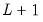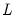breakspec
Display the breakpoint specification results for an equation estimated using breakls.
Syntax
eq_name.breakspec
Options

 p Print basic estimation results.
Examples
equation eq1.breakls m1 c unemp
eq1.breakspec(p)
displays and prints the breakpoint determination results for the equation EQ1 estimated using Bai-Perron sequentialversustests to determine the optimal breaks.
Cross-references
See “Least Squares with Breakpoints” for discussion.